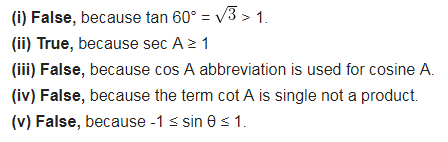# State whether the following statements are true or false. Justify your answer

State whether the following statements are true or false. Justify your answer.
(i) The value of tan A is always less than 1.
(ii) sec A =12/5 for some value of angle A.
(iii) cos A is the abbreviation used for the cosecant of angle A.
(iv) cot A is the product of cot and A.
(v) sin θ = 4/3 for some angle.